# Acid

The barrel is 199 liters of 38% acid. How many percent acids occur when 61 liters revoke and replace with the same amount of water?

### Correct answer:

acid concentration =  26.4 %

### Step-by-step explanation:

$c=\frac{199-61}{199}\cdot 38=26.4\mathrm{%}$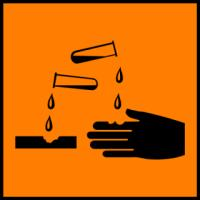We will be pleased if You send us any improvements to this math problem. Thank you!Tips to related online calculators
Our percentage calculator will help you quickly calculate various typical tasks with percentages.
Check out our ratio calculator.
Do you have a linear equation or system of equations and looking for its solution? Or do you have a quadratic equation?

## Related math problems and questions:

• Acids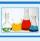70% acid made from the same acid of two different concentrations. Amount weaker acid to the stronger acid is in ratio 2:1. What was the concentration of the weaker acid if stronger had 91% concentration?
• Acid solutionBy adding 250 grams of a 96% solution of sulfuric acid to its 3% solution its initial concentration was changed to 25%. How many grams of 3% of the acid were used for dilution?
• Acid evaporationHow many kilograms of water do we have to evaporate from 100 kg of 32% acid to make it 80% concentration?
• ConcentrationHow many grams of 65% and 50% acid is to be mixed to give 240 g acid with a concentration of 60%?
• Solutions, mixturesHow many liters of 70% solution we must add to 5 liters of 30% solution to give us a 60% solution?
• AlcoholHow many 55% alcohol we need to pour into 14 liters 75% alcohol to get p3% alcohol? How many 65% alcohol we get?
• Pouring alcohol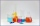100 liters of alcohol has 70% How many liters of water need to be added to have 60% alcohol?
• SolutionWhat amount of 80% solution and what amount of water is needed to mix in order to obtain two liters of 60% solution of this mixture?
• Alcohol mixtureThree liters of 96 percent alcohol set up a certain amount of distilled water to form 54-percent alcohol. How many liters of distilled water was used?
• A 36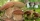A 36 g sample of the substance contains 91% water. After drying, the weight of the sample was reduced to 18 g. What percentage of water is now in the sample?
• SolutionsHow much 60% solution and how much 35% solution is needed to create 100 l of 40% solution?
• Alcohol 2Two types of alcohol one 63% and second 75% give 20 liters of 69% alcohol. How many liters of each type are in the mixture?
• Peroxide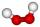How much distilled water (in liters) must pharmacists pour into 300 ml of 23.6% solution of hydrogen peroxide to get 2.7% solution to gargle?
• CoffeeIn-stock are three kinds of branded coffee prices: I. Kind. .. .. .205 Kc/kg II. Kind. .. .. .274 Kc/kg III. Kind. .. .. 168 Kc/kg Mixing these three species in the ratio 8:5:6 create a mixture. What will be the price of 100 grams of this mixture?
• Mushrooms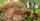Fresh mushrooms contain 90% water, dried only 12%. How many kilograms of fresh mushrooms are needed for 5kg dried?
• Solutions, mixturesWe have 2 liters of 20% solution available. How much 70% solution do we need to add to it to get a 30% solution?
• Sea waterSeawater contains about 4.3% salt. How many dm3 of distilled water we must pour into 5 dm3 of sea water to get water with 1.8% salt?